## 2. Energy of the classical harmonic oscillator.

If a particle with mass m, removed from its position of rest O, is subject to an elastic force f, that is a force proportional to the displacement Δr from O and opposite to the displacement,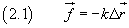the displacement r of the particle from O is given as a function of time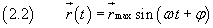A particle having such kind of motion is called harmonic oscillator.

In order to obtain the (2.2), we choose a reference system with origin O and abscissa axis coincident with the displacement. In this system the (2.1) is expressed by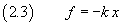From the second law of dynamics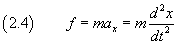so the (2.3) may be written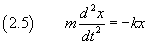We let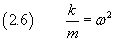therefore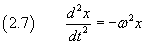The solution of the differential equation (2.7) must be a function x(t) such that its second derivative with respect to the time t coincides with the opposite of the function itself times ω2. Such function can be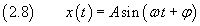Indeed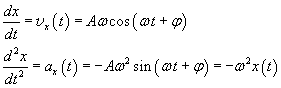In (2.8) the quantity A, that represents the greatest absolute value of the displacement x(t), is called amplitude.

The quantity φ, called phase constant, represents the argument of the sine at the time t=0.

If the motion is such that x(0)=0 and vx(0)=vMAX, the phase constant is 0 and the (2.8) may be more simply written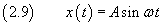The physical meaning of the quantity ω may be understood if we call T the time elapsed between two successive maxima of x(t) in the (2.9). In fact this function has its maxima A when sin ωt=1, that is when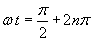Therefore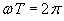that is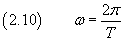The quantity T is called period and may be defined as the the time for one complete oscillation. The reciprocal of T, called frequency and usually denoted by ν (Greek nu), is the number of oscillations that are completed each second: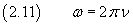The quantity ω, said angular frequency, is proportional to the frequency and gives identical physical informations.

With the definition (2.6) of ω, the (2.3) can be written as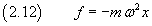The work of an elastic force f when it moves a particle with mass m along a path dx parallel to the force itself is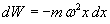so the potential energy of the particle at the position x is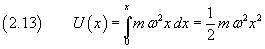The total mechanical energy of the harmonic oscillator is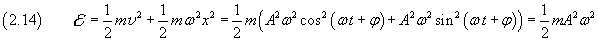The total mechanical energy of the harmonic oscillator is constant.